Sismanoglu, S., Akalin, M. K., Akalin, G. O., and Topak, F. (2023). “Effective removal of cationic dyes from aqueous solutions by using black cumin (Nigella sativa) seed pulp and biochar,” BioResources 18(2), 3414-3439.

#### Abstract

Black cumin seed pulp (C), as well as biochar (CC) produced via pyrolysis of black cumin seed pulp were used to remove methylene violet 2B (MV) and basic yellow 28 (BY28) from aqueous solution. Adsorption isotherms and kinetics were applied at 10, 25, and 35 °C. The adsorption of methylene violet 2B and basic yellow 28 on the black cumin seed pulp and biochar surface was exothermic; the heat of adsorption values were lower than 0. The adsorption capacities of BY28-C, BY28-CC, MV-C, and MV-CC were 212.8, 625, 164, and 909 mg g-1 at 25 °C, respectively. The adsorption of black cumin seed pulp and biochar data were examined with the Freundlich, Langmuir, Temkin, Dubinin-Radushkevich (D-R), and Flory-Huggins (F-H) isotherm models. The kinetics of the adsorption were fitted to the pseudo first-order and pseudo second order equations. The pseudo second order equation gave a better fit than the pseudo first-order equation.

Effective Removal of Cationic Dyes from Aqueous Solutions by Using Black Cumin (Nigella sativa) Seed Pulp and Biochar

Sedef Sismanoglu,a Mehmet Kuddusi Akalin,b,* Gulen Oytun Akalin,c and Fatıma Topak b

Black cumin seed pulp (C), as well as biochar (CC) produced via pyrolysis of black cumin seed pulp were used to remove methylene violet 2B (MV) and basic yellow 28 (BY28) from aqueous solution. Adsorption isotherms and kinetics were applied at 10, 25, and 35 °C. The adsorption of methylene violet 2B and basic yellow 28 on the black cumin seed pulp and biochar surface was exothermic; the heat of adsorption values were lower than 0. The adsorption capacities of BY28-C, BY28-CC, MV-C, and MV-CC were 212.8, 625, 164, and 909 mg g-1 at 25 °C, respectively. The adsorption of black cumin seed pulp and biochar data were examined with the Freundlich, Langmuir, Temkin, Dubinin-Radushkevich (D-R), and Flory-Huggins (F-H) isotherm models. The kinetics of the adsorption were fitted to the pseudo first-order and pseudo second order equations. The pseudo second order equation gave a better fit than the pseudo first-order equation.

DOI: 10.15376/biores.18.2.3414-3439

Keywords: Black cumin seed pulp; Biochar, BY28; MV; Adsorption

Contact information: a: Department of Chemistry, Karabuk University, 78050, Karabuk, Turkey; b: Department of Environmental Engineering, Karabuk University, 78050, Karabuk, Turkey; c: Medical Laboratory Program, Department of Medical Services and Techniques, Vocational School of Health Service, Aksaray University, 68100, Aksaray, Turkey;

* Corresponding author: mehmetakalin@karabuk.edu.tr

INTRODUCTION

Synthetic dyes are potential water pollutants that are widely used by the textile industry; they cause problems because of their toxicity and their coloring (Han et al. 2015; Singh et al. 2022). Adsorption is an effective way to remove dyes from wastewaters, and activated carbon is widely used for this purpose. However, the activation process increases the cost and energy consumption of the process. Chemical activation is widely used to produce activated carbon from biomass. However, using chemicals for the activation increases the cost of the process and causes hazardous effects to the environment (Choi et al. 2019; Vigneshwaran et al. 2021). Therefore, using an adsorbent that is abundant in nature, or a waste or a by-product of a process becomes important. Biochar is carbon-rich material that is obtained via thermochemical conversion of biomass. Despite having low adsorption capacities, biochar is a potential alternative to activated carbon in water treatment due to its low cost and not using chemicals for the activation (Zazycki et al. 2018; Choi et al. 2019). Agricultural byproducts that have low economic value and biochar produced from those materials are good alternative adsorbents for the removal of dyes from wastewater.

Black cumin is used in the treatment of cancer, diabetes, asthma, and kidney diseases due to the different active pharmaceutical components in its content. It is grown in Southwest Asia and Eastern Mediterranean countries (Zent 2019; Yimer et al. 2019; Can 2020). In Turkey, black cumin is grown in provinces such as Mersin, Gaziantep, Isparta, Burdur, Uşak, and Bursa (Koşar and Özel 2018; Can 2020). While black cumin seed production in Turkey was 161 tons in 2012 (2299 decares), it increased to 3603 tons in 2019 (37085 decares). When considering the foreign trade data of black cumin, it can be seen that 2647.5 tons of black cumin seed (\$2.53 million) was imported in the same year, equal to the export of 592.4 tons (\$1.23 million) of black seed in 2019 (Can 2020). Black cumin is used as a health supplement and condiment, as well as spice in the food industry, and the pulp is used as a filler in the production of environmentally friendly polymer composites (Yimer et al. 2019; Sismanoglu et al. 2022). It is used as feed in animal nutrition in order to prevent environmental pollution and create added value from the pulp part that is released during the production of black seed oil (Zent 2019).

Compared with other studies with basic yellow 28 (BY28), the adsorbent (GAC-HB) was added to 200 mL of basic yellow 28 dye solution on coconut shell charcoal granular activated carbon. Different studies were conducted on sorbent dose, initial dye concentration, solution pH 9, GAC-HB dose 0.8 g/L, and contact for 2 h. The optimum conditions for BY28 were determined. The Langmuir qmax value was 769 mg/g (Dao et al. 2013). Activated carbon produced from Persea americana nut (PAN) was used with V= 50 mL BY 28 (100 mg/L) in at ambient temperature and Langmuir maximum uptake (qmax) at 0.2 g/L dosage was 325 mg/g (Regti et al. 2017). The adsorbent is related to the adsorption of BY28 to low-cost natural red clay (NRC). For adsorption studies, contact time, initial concentration, pH, adsorbent mass, and temperature were studied as 1 to 180 min, 50 to 300 mg/L, 3-10, 0.5 to 10 g/L, and 25 to 40 °C, respectively. Langmuir maximum monolayer adsorption capacity qmax was 370 mg/g. The adsorption of basic yellow 28 dye on adsorbent Ca-bentonite was investigated at pH 7.0 for temperatures of 20 and 40 °C. The Langmuir maximum monolayer adsorption capacity (qmax) of Ca-bentonite was determined as 94.3 mg/g at 20 °C and 99.0 mg/g at 40 °C (Kalpaklı et al. 2014).

In this study, black cumin seed pulp, which has a low economical value, and biochar, which was produced via pyrolysis of black cumin seed pulp, were used as low-cost adsorbents for the removal of methyl violet 2B and basic yellow 28. The characterization of the adsorbent was performed before and after adsorption by FTIR/ATR, TGA, and SEM. The removal of methylene violet 2B (MV) and basic yellow 28 (BY28) were tested, and different kinetic and adsorption isotherms models were applied.

EXPERIMENTAL

Feedstock and Chemicals

Black cumin (Nigella sativa) seed pulp was obtained at no cost from Bağdat Spices Co., Ankara, Turkey, and it was used as received. Methyl violet 2B (CAS number: 8004-87-3, molecular weight: 393.95 g/mol) and basic yellow 28 (CAS number 54060-92-3, molecular weight: 433.52 g/mol) were used in the adsorption experiments. Basic yellow 28, which is used in textiles with azo group, ionizes in its aqueous solutions and forms positively charged ions and is therefore a cationic dye (Slimani et al. 2014; Benkaddour et al. 2020). Methyl violet 2B, known as triphenylmethane dyes because it has 3 aryl groups attached to a nitrogen atom interacting with one or two methyl groups, is a basic dye due to the positive charge on the amino group, and its aqueous solutions are purple while the powder is dark green (Bonetto et al. 2015; Dahri et al. 2015). The chemical formulas of active pharmaceutical ingredients in black cumin thymol and the molecular structures of BY28 and MV 2B are shown in Figs. 1 and 2, respectively.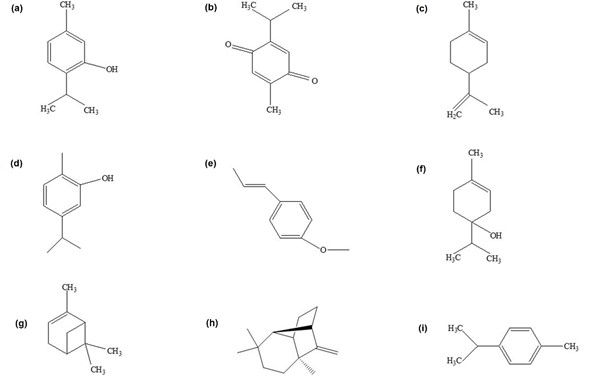Fig. 1. Chemical formulas of active pharmaceutical ingredients in black cumin thymol (a), thymoquinone (b), limonene (c), carvacrol (d), t-anethole benzene (e), 4-terpineol (f), α-pinene (g), longifolene (h), p-simen (i) (Sismanoglu et al. 2022)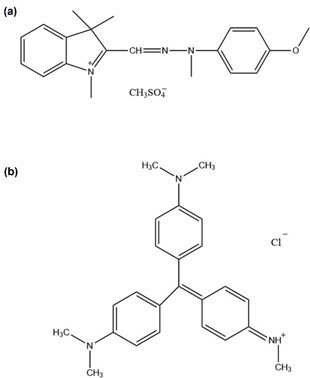Fig. 2. Molecular structure of BY28 (a), MV (b)

Production of Biochar

The biochar was produced via pyrolysis of black cumin seed pulp at 500 °C with a heating rate of 10 °C/min. The pyrolysis experiments were carried out in a 250 mL stainless steel fixed bed reactor in which continuous flow of nitrogen gas was used to provide an inert atmosphere. In the pyrolysis experiment, 10 g of black cumin seeds (dry basis) was placed in the reactor. Before heating the reactor to 500 °C with a heating rate of 10 °C/min, the air inside the reactor was purged with nitrogen flow for 15 min. When the temperature reached to 500 °C, the reactor was held at that temperature for 1 h and then the heating was stopped. After cooling of the reactor to room temperature, the reactor was opened, and biochar was taken from inside the reactor. No further treatment was applied to the biochar before the adsorption experiments.

The changes of adsorbates and adsorbents surfaces before and after adsorption were characterized by FTIR and SEM. In addition, thermal behavior before and after adsorption was analyzed by TGA. FTIR/ATR analysis was performed on the Alpha Bruker FTIR spectrometer, in the range of 4000 to 500 cm-1, in ATR mode. SEM micrographs were taken by Carl Zeiss Ultra Plus Gemini field emission scanning electron microscope (FESEM) (Oberkochen, Germany) at a X20000 magnification. Before the experiment, the powders were covered with gold sputtering for 15 min. Thermal analysis of adsorbents and adsorbates was carried out using the Hitachi STA 7300 Thermogravimetric Analyzer in the temperature range of 25 to 600 °C in an inert nitrogen atmosphere at a heating rate of 10 ˚C/min and a nitrogen gas flow of 20 mL/min.

Solutions of BY28 150, 100, 75, 50, 25 ppm and MV 200, 150, 100, 50, 25 ppm concentrations were made in a shaking water bath for 10, 25, 35 °C at certain intervals for a total of 60 min.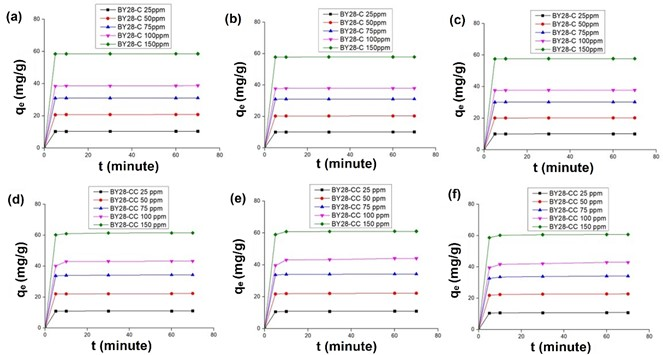Fig. 3. Graphs of qe (mg/g) vs. time of BY28-C at 10 °C (a), 25 °C (b), 35 °C (c); BY28-CC at 10 °C (d), 25 °C (e), 35 °C (f)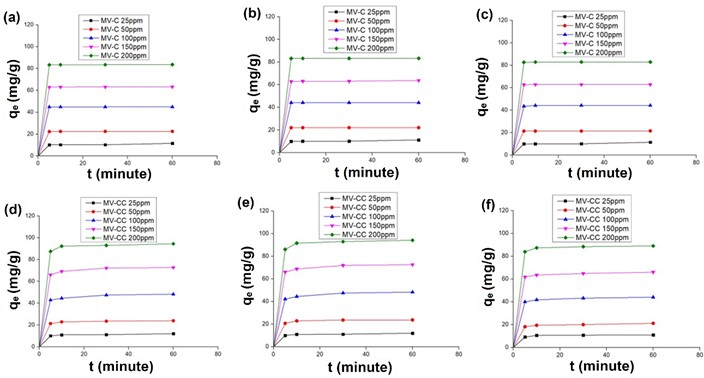Fig. 4. Graphs of qe (mg/g) vs. time of MV-C at 10 °C (a), 25 °C (b), 35 °C (c); MV-CC at 10 °C (d), 25 °C (e), 35 °C (f)

The equilibrium time of adsorption was chosen as 30 min for BY28 (Fig. 3) and 60 min for MV (Fig. 4) from the qe vs. t plot. A total of 10 mL of dye concentrate, 0.02 g of black cumin, and black cumin char were used. The dose rate was 2 g/L. The pH for BY28 was 5.5 to 6, while the pH of MV was 5 to 6. The amount of dye collected on unit absorbent, qe (mg/g), calculated as follows,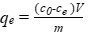(1)

where ce is the dye concentration remaining in solution after adsorption (mg/L), c0 is the initial concentration of dye (mg/L), V is the total dye volume (L), and m is the adsorbent amount (g).

For adsorption isotherms, Freundlich, Langmuir, Temkin, Dubinin and Radushkevich (D-R), and Flory-Huggins (F-H) isotherms were used. In thermodynamic calculations, the standard Gibb’s energy was calculated with the following equation (Malkoc and Nuhoglu 2007),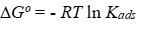(2)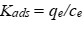(3)

where Kads (L/g) is the standard thermodynamic equilibrium constant. Using this equilibrium constant, with the following equation,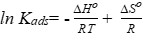(4)

where lnKads versus 1/T enthalpy from the slope of the plot provided the heat of reaction (ΔHo kj/mol), and the entropy (ΔSo j/molK) was obtained from the intercept with the vertical axis (Malkoc and Nuhoglu 2007; Rahchamani et al. 2011).

Isotherms

Freundlich

According to this isotherm, the adsorption areas on the surface of an adsorbent are heterogeneous; it is composed of different types of adsorption sites. The Freundlich isotherm equation in linear form is as follows,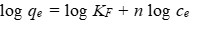(5)

where KF is the adsorption capacity (L/mg), and n is the adsorption density (Hakan Duran et al. 2019). When n<1, adsorption is favorable (Reed and Cline 1994).

Langmuir

This isotherm assumes that there are trapping points on the adsorbent surface. Assuming that each holding point will adsorb a dye molecule, the layer formed has a thickness of one molecule. In equilibrium, it reaches the maximum adsorption capacity and the surface is covered with a monolayer (Duran et al. 2019), as follows,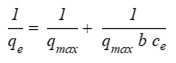(6)

Temkin

According to this isotherm, the heat of adsorption decreases linearly with the coating of the surface due to the interactions between the adsorbed dye molecules, and the participation of this heat during the adsorption will be either endothermic or exothermic (Malkoc and Nuhoglu 2007).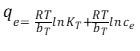(7)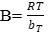(8)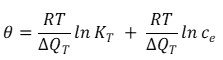(9)

where bT is the Temkin constant (kj/molK), B is the adsorption potential (energy) related (kJ/mol), KT is the Temkin isotherm constant (L/mg), R is the gas constant (J/molK), and θ is the ratio of the components adsorbed on the surface of the adsorbent.

The equilibrium amount (qe mg/g) of adsorbed dyes on the surface of the adsorbent is divided by the monolayer adsorption capacity (qmax mg/g) from Langmuir curves. ΔQ is the adsorption energy (kJ/mol). Assuming that the process is carried out under a closed system, the heat value ΔQ is almost the same as ΔH. As a thermodynamic formula, ΔQT = – ΔH. The ΔH value can be defined as follows: ΔH > 0 kJ/mol are endothermic processes, and ΔH < 0 kJ/mol are exothermic processes (Pursell et al. 2011; Kireç et al. 2021).

According to this isotherm, a retained layer exhibits multi-layer character, and the following expression is used to calculate the adsorption energy of the adsorption system (Savran et al. 2017),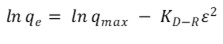(10)

where KD-R is the adsorption energy corresponding constan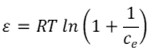t (mol2kJ2), and ε is the Pollanyi constant, calculated with , and KD-R is the adsorption energy calculated using this formula by using the relevant constant, as shown below.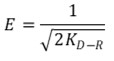(11)

It has been stated that if this energy is within the range 8 kJ/mol < E < 16 kJ/mol, the adsorption mechanism is ionic exchange, if E < 8 kJ/mol, the adsorption mechanism is physical adsorption, and if E > 16 kJ/mol, the adsorption mechanism is diffusion (Savran et al. 2017).

Flory-Huggins (F-H)

While deriving the degree of coating of the surface by the dye on the adsorbent, this isotherm informs about the feasibility of the adsorption mechanism, that is, whether it is spontaneous or not (Saadi et al. 2015),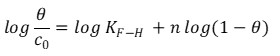(12)

where θ is the degree of surface coverage, KF-H is the isotherm constant, and n is the number of ions at sorption sites. Equation 13 defines the degree of surface coverage.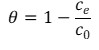(13)

The Gibb’s energy is calculated using the KF-H constant.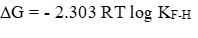(14)

If ΔG (kj/mol) < 0, the adsorption mechanism will be spontaneous; if ΔG (kj/mol) > 0, the adsorption mechanism will not be spontaneous (Saadi et al. 2015).

Kinetics

Pseudo First Order Kinetic Model

The pseudo-first order kinetic model was given by Lagergren (1898) for the adsorption of solid/liquid systems and its formula is as follows,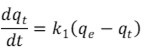(15)

where k1 is the first order adsorption rate constant (min-1), qt is the amount of substance adsorbed at time t (mgg-1), and qe is the amount of substance adsorbed (mgg-1) at equilibrium. The equation with integral and boundary conditions applied is given by: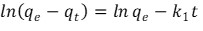(16)

when plotting ln(qe-qt) versus t, the slope is k1 (1/time).

Pseudo Second Order Kinetic Model

The basis of the second order kinetic model is based on the adsorption capacity of the solid phase (Hubbe et al. 2019), as follows,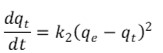(17)

where k2 is the second order adsorption constant (g mg-1 min-1). At t=0, qt=0. At t=t, qt= qt boundary conditions are applied and equality integral is taken, as follows.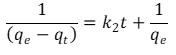(18)

According to McKay and Ho the linearized form of the equation (Ho et al. 1999) can be expressed as,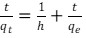(19)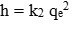(20)

where h (mg g-1 min-1) gives the initial sorption rate. If the graph of t/qt versus t is drawn, qe and k2 are calculated from the slope and intercept points (Azizian 2004).

RESULTS AND DISCUSSION

FTIR/ATR Analysis Results

When the FTIR/ATR of BY28 and MV dyestuffs are examined, N-H stretching peaks in the range of 3500 to 3000 cm-1 and in-plane asymmetric stretching peaks of the CH2 and CH groups are observed at 1600 cm-1 (Erdik 1998). The small peak seen at 2978 cm-1 in black seed can be attributed to the -CH band and the N-H peak (Erdik 1998; Carrión-Prieto et al. 2017; Thabede and Shooto 2022). The peak at 2915 cm-1 shows the -CH stretching in the -CH3 and -CH2 groups (Thabede and Shooto 2022). In the FTIR/ATR graph of black cumin char, the N-H peak disappeared after pyrolysis. The peaks between 2400 to 2300 cm-1 in black seed and char are C≡N stretch absorbance peaks (Durak et al. 2019). When BY28-C and black seed FTIR/ATR were compared, the intensity of the N-H peak between 3200 to 2800 cm-1 increased with the effect of the functional groups of the BY28 dyestuff. In the BY28-CC and char graphs, a new double peak is formed between 3200 to 2800 cm-1, and the NH groups of the BY28 dyestuff are attached to the surface of the char. In addition, the C≡N peaks in both black seed and char intensified as the CH=N structure in BY28 penetrated the structure of black cumin and char. In the graph of BY28-CC and char FTIR/ATR, a new peak occurred in BY28-CC due to the in-plane asymmetric stretching of the CH2 and CH groups at 1600 cm-1 in the structure of BY28. As with the BY28-CC, the MV-CC FTIR/ATR results are almost identical. After the adsorption of black cumin with BY28, the intensity of the peak at 1600 cm-1 increased. After adsorption of black seed with MV, the N-H peak of black cumin between 3200 to 2800 cm-1 became sharper with the penetration of the MV dyestuff. In addition, when looking at the groups present in the black cumin structure (Fig. 1); C-O tension is seen at 1064 cm-1, and the aromatic extra-ring C-H bend and alkene C-H bend functional group wavelength is 668 cm-1 (Nandiyanto et al. 2019). In the BY28 dyestuff structure, it was observed that the C-S tension of CH3OSO3 and the C-H and C-O groups of black cumin increased the peak intensities at 1064 cm-1 and 668 cm-1 more than the MV dye. From this, it was thought that BY28 was more adsorbed in black cumin.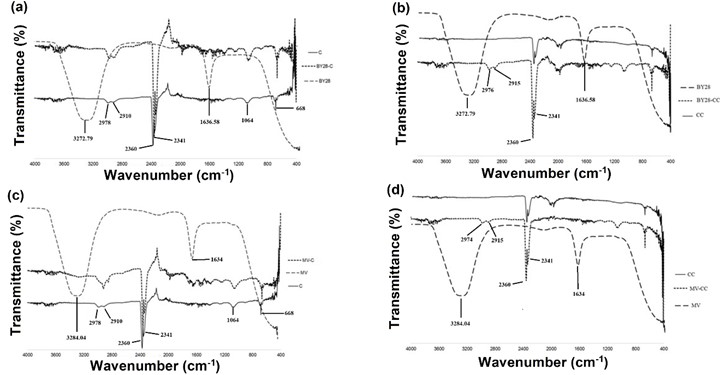Fig. 5. FTIR/ATR spectrums of BY28-C (a), BY28-CC (b), MV-C (c), MV-CC (d)

SEM Analysis Results

Black cumin has a slotted and mounded structure, while char has a scaly and channeled structure. After the adsorption of BY28 on black cumin (Fig. 6a), the BY28 dye molecules settled into the slits and covered the surface, and the structure became smoother.

After the adsorption of MV on black cumin (Fig. 6c), a wavy structure was observed in which the dye molecules completely covered the surface, and the slits were closed. After the adsorption of char with BY28 (Fig. 6b), the surface porosity of the char increased, and the dyestuff was stuck on the surface. After adsorption with MV, channel depths were reduced by MV molecules, and its scaly structure was covered by MV (Fig. 6d). A flatter structure was formed in the adsorption of char with MV (Fig. 6d).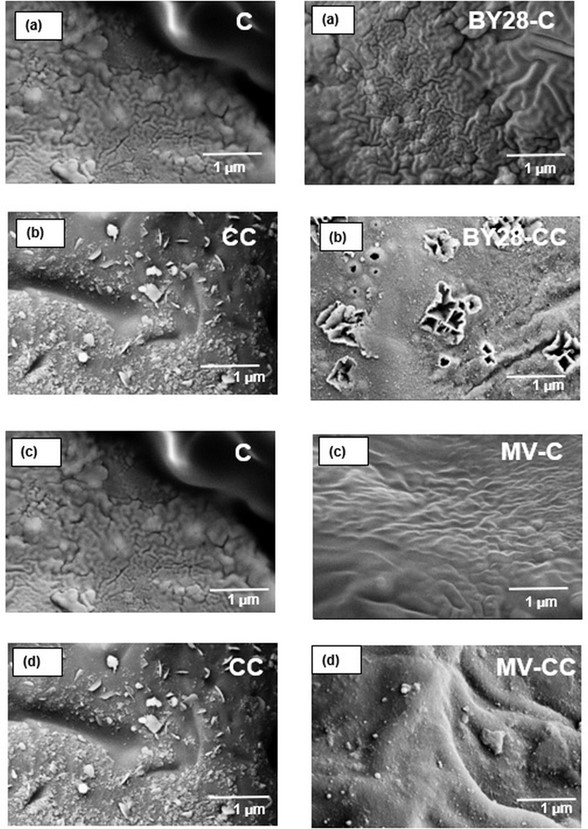Fig. 6. Surface morphologies of BY28-C (a), BY28-CC (b), MV-C (c), MV-CC (d)

TGA Results

TGA curves for black cumin seeds and biochars before and after adsorption are shown in Fig. 7. The results were similar for black cumin seeds before and after adsorption. The evaporation of moisture was observed with the weight loss up to 135 °C. The further weight loss until 600 °C includes the thermal decomposition of hemicellulose, cellulose, and lignin. The thermal degradation of biochars was roughly similar. The evaporation of absorbed water in biochars was observed with the weight loss up to 200 °C. The weight loss at between 200 and 450 °C can be attributed to the degradation of cellulose, hemicellulose, and other volatile components. The weight loss above that temperature reflects the degradation of residual lignin (Choudhary et al. 2020; Sismanoglu et al. 2022; Deng et al. 2022). When the thermal behavior graphs of the adsorption of dyestuffs with pure black cumin and pure black cumin in Figs. 7a and 7c are examined, the stages seen in the graphs are due to the impurities in the black cumin structure. In Figs. 7b and 7d, it can be seen that the impurities in black cumin decreased with pyrolysis and the temperature resistance increased in the thermal behavior graphs of the adsorption of char and char and with dyestuffs. It was observed that the weight loss (%) in the TGA curve of the adsorption experiments performed with black cumin was higher and the thermal resistance decreased accordingly. When the experiments with char were examined, it was seen that the thermal resistance values before and after adsorption were close to each other and the thermal resistance was slightly higher for both dyes after adsorption at high temperatures. These results are also consistent with the qmax values in Tables 1 and 2.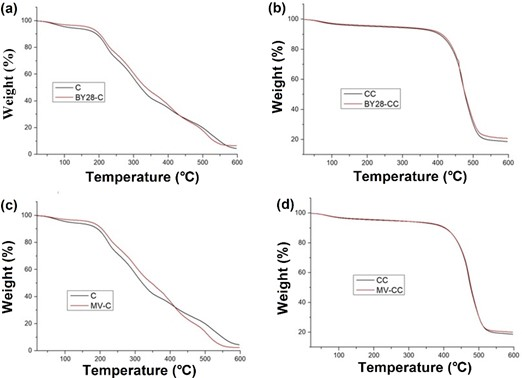Fig. 7. TGA of BY28-C (a), BY28-CC (b), MV-C (c), MV-CC (d)

Shapes of the Isotherms

The ce graphs (Figs. 8 and 9) drawn against qe from the adsorption isotherm curve of both dyestuffs on the adsorbents conformed to the Giles isotherm L type. Looking at the graphs, it becomes difficult for the dye molecules to find empty space as the empty spaces on the first curve (layer) part of the adsorbent (black seed and char) are filled rapidly. Therefore, the second curve (layer) is formed. This means that a new monolayer is formed on top of the first layer (Giles et al. 1960). Thus, the dye molecules, rather than the solvent molecules, are more aggressively directed towards the adsorbent.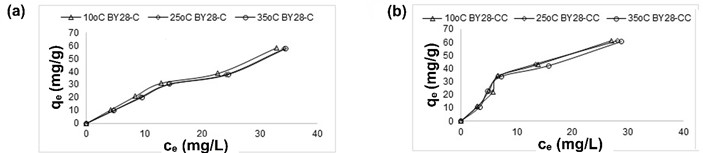Fig. 8. Graphs of qe (mg/g) vs. ce of BY28-C at 10, 25, and 35 °C (a); BY28-CC at 10, 25, and 35 °C (b)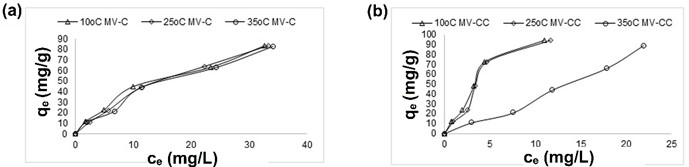Fig. 9. Graphs of qe (mg/g) vs. ce of MV-C at 10, 25, and 35 °C (a); MV-CC at 10, 25, and 35 °C (b)

As shown in Tables 1 and 2, the qmax value of BY28-C for all three temperatures was quite high compared to MV-C. The qmax values of BY28-C and MV-C at 25 °C were 212.8 mg/g and 164 mg/g, respectively. When compared with other temperature values, it can be seen that the maximum adsorption capacity (qmax) of black cumin in the highest monolayer was 25 °C. The qmax values of black cumin char of BY28 and MV (BY28-CC, MV-CC) at 25 °C were 625 mg/g and 909 mg/g, respectively. The qmax value of the char at 25 °C was higher than the values at other temperatures. According to Tables 1 and 2, the qmax of the BY28-C at 25 °C was higher when the MV-C was compared with the BY28-C. Likewise, when MV-CC and BY28-CC were compared according to Tables 1 and 2, the qmax value of MV-CC at 25 °C was higher. As a result, while the qmax (mg/g) data of the adsorption capacity in the Langmuir model preferred BY28 in the raw form of black cumin; it showed that the one in the char state preferred MV. While the compatibility of BY28 to the Langmuir isotherm (R2) of adsorption on both adsorbent at three different temperatures was between 98% and 99% for black cumin, it was between 88% and 96% for black cumin-char. The suitability of MV to Langmuir isotherm (R2) was between 96% and 99.8% for black cumin; it was between 97% and 99% for black cumin-char.

When the Temkin isotherm is applied for BY28-C, MV-C, BY28-CC, and MV-CC, the adsorption type of BY28 and MV on adsorbents is physical adsorption, since B, that is, the adsorption potential (energy) in the first equation of Temkin, is lower than 8 kJ/mol (Tables 1 and 2). The ΔQ adsorption energy is almost the opposite sign of the ΔH adsorption heat value result. Using the second equation of the Temkin, it was calculated that the adsorption heat of BY28 and MV dyestuffs on both adsorbent is exothermic. For black cumin and char, in which basic yellow 28 was used as dyestuff, the equivalence of the Temkin isotherm equation 7 (R2) was between 92% and 93%, while it was between 93% and 99% for black cumin and char, respectively. When BY28 was used, the equivalence of the Temkin isotherm equation 9 (R2) was between 93% and 95% for black cumin, while it was between 97% and 98% for black cumin char. The equivalence of MV adsorption on both adsorbent at three different temperatures to the Temkin isotherm equation 7 (R2) is between 88% and 96% for black cumin, while it is between 86% and 94% for black cumin char. The equivalence conformity to the Temkin isotherm equation 9 (R2) is between 91% and 96% for black cumin, and 86% to 94% for black cumin char.

Dubinin and Radushkevich (D-R) adsorption isotherm results, as shown in Tables 1 and 2 qmax, that is, the maximum saturation capacity for BY28-C is 41 to 42 mg/g; 42 to 53 mg/g for BY28-CC; 51 to 56 mg/g for MV-C; For MV-CC, it is 57 to 67 mg/g. Since the calculated adsorption mechanism energy (E) of both dyes on the adsorbents is lower than 8 kJ/mol, it is seen that physical adsorption takes place. This value is compatible with the B value in Temkin’s first equation. The conformity of BY28 to the D-R isotherm of adsorption at three different temperatures on both adsorbent (R2) is between 85% and 86% for black cumin, while it is between 93% and 97% for black cumin-char. The suitability of adsorption of MV on both adsorbent at three different temperatures to the D-R isotherm (R2) is between 71% and 81% for black cumin, while it is between 75% and 82% for black cumin-char.

From the Flory-Huggins (F-H) adsorption isotherm results for BY28-C, BY28-CC, MV-C, and MV-CC, (Tables 1 and 2), logKF-H was positive as a result of adsorption of both dyes on black cumin and char, and the adsorption reaction energy was calculated from Eq. 14 as ΔG kj/mol < 0. The adsorption of both dyes on black cumin and black cumin-char was spontaneous.

Table 1. Isotherms Data of BY28-C and BY28-CC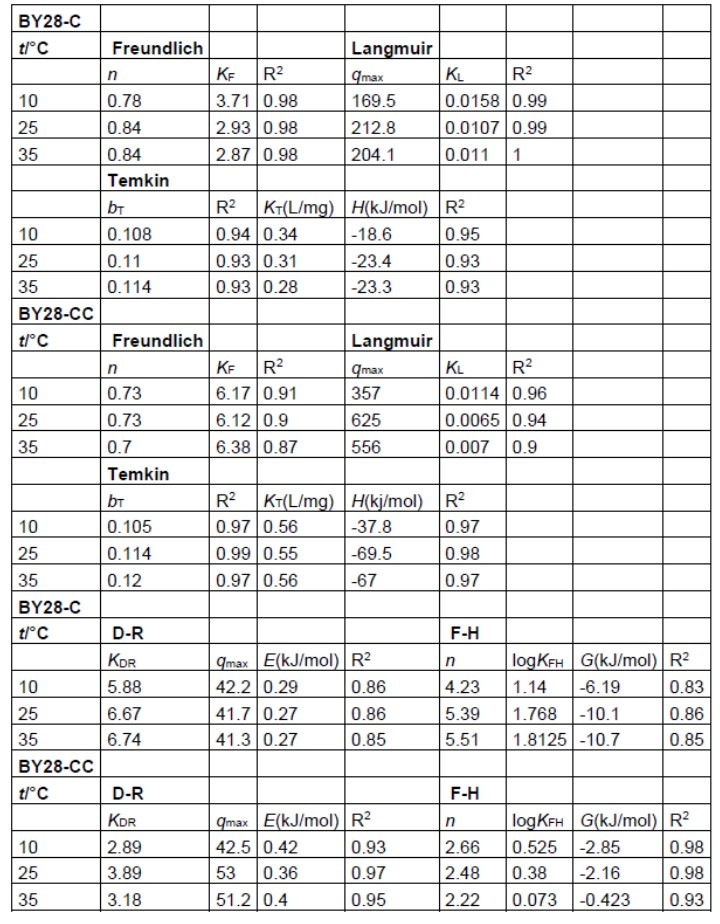Table 2. Isotherms Data of MV-C and MV-CC